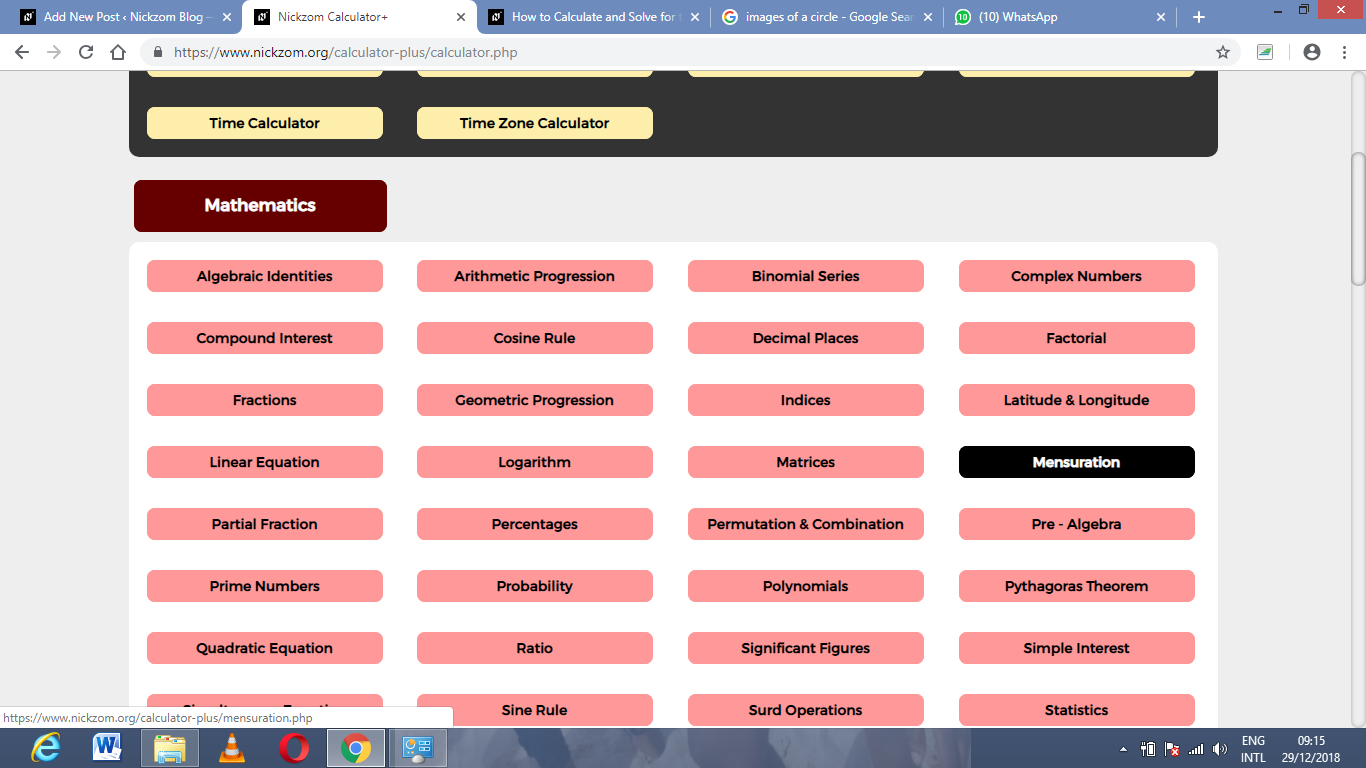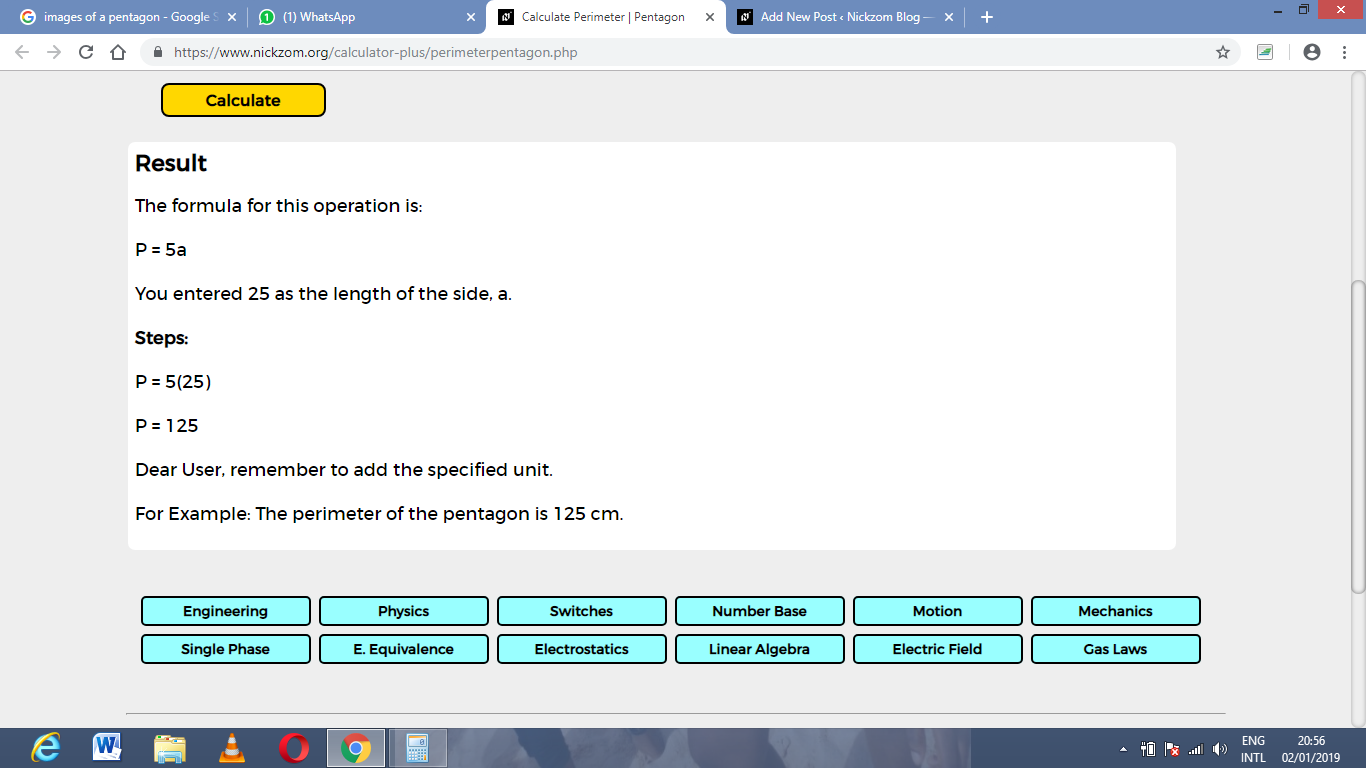# How to Calculate and Solve for the Length of a Side and Perimeter of a Pentagon | Nickzom CalculatorThe image above is a pentagon.

To compute the perimeter of a pentagon, one essential parameter is needed and this parameter is length of side (a).

The formula for calculating the perimeter of a pentagon:

P = 5a

Where;
P = Perimeter of the pentagon
a = length of side

Let’s solve an example;
Find the perimeter of a pentagon when the length of side (a) is 25 cm.

This implies that;
a = length of side = 25 cm

P = 5a
P = 5 x 25
P = 125

Therefore, the perimeter of the pentagon is 125 cm.

Calculating the length of side using the perimeter of the pentagon.

a = P / 5

Where;
a = Length of side
P = Perimeter of the pentagon

Let’s solve an example;
Given that the area of the pentagon is 250 cm. Find the length of side?

This implies that;
P = Perimeter of the pentagon = 250 cm

a = P / 5
a = 250 / 5
a = 50

Therefore, the length of side (a) is 50 cm.

Nickzom Calculator – The Calculator Encyclopedia is capable of calculating the perimeter of a pentagon.

To get the answer and workings of the perimeter of a pentagon using the Nickzom Calculator – The Calculator Encyclopedia. First, you need to obtain the app.

You can get this app via any of these means:

To get access to the professional version via web, you need to register and subscribe for NGN 1,500 per annum to have utter access to all functionalities.
You can also try the demo version via https://www.nickzom.org/calculator

Once, you have obtained the calculator encyclopedia app, proceed to the Calculator Map, then click on Mensuration under the Mathematics sectionNow, click on Perimeter of a Pentagon under MensurationThe screenshot below displays the page or activity to enter your value, to get the answer for the perimeter of a pentagon according to the respective parameter which is the length of side (a).Now, enter the values appropriately and accordingly for the parameter as required by the example above where the length of side (a) is 25 cm.Finally, Click on CalculateAs you can see from the screenshot above, Nickzom Calculator – The Calculator Encyclopedia solves for the perimeter of a pentagon and presents the formula, workings and steps too.